# Wavelet Analysis

In this demo we will learn to apply wavelet analysis. This is a great way to visualize the variability in your time series.

# Topics Covered

The topics covered in this assignment are
1. Simple smoothing revisited
2. Bandpass filtering
3. The Morse wavelets
4. Choosing the wavelet parameters
5. Computing the wavelet frequency array with morsespec
6. Computing the wavelet transform with wavetrans
7. Plotting the wavelet transform with wavespecplot
8. Computing the wavelets themselves with morsewave
9. The wiavelet scaling laws
10. The wavelet transforms of boxcars and sinusoids
11. Why you should always look at the wavelets
12. Bivariate and rotary wavelet transforms

# Simple Smoothing Revisited

First we will review simple smoothing of our data, which we first encountered on day one, from another point of view.
Generally, the goal of smoothing is to remove high frequencies. This is why we often use the term lowpass, because we want to design a filter through which will let the low frequencies pass through, but which will reject the high ones.
Let's see how the boxcar or rectangle function performs as a lowpass filter. Whereas, as we have seen, the Fourier transform of a Gaussian is also a Gaussian, the Fourier transform of a boxcar is a very bouncy function.
t=[1:1001]';t=t-mean(t);
g=zeros(size(t));
g(abs(t)<10)=1/19;%this is a 19-point boxcar
clf
subplot(2,1,1),plot(t,g),xlabel('Time')
subplot(2,1,2),plot(abs(fft(g))),xlabel('Frequency'),axis tight
Notice that rather than decaying to zero, the curve hits zero and bounces back repeatedly. This has undesireable consequences, as we will see.
Now we will use that boxcar to smooth a dataset. For simplicity, we will use a dataset consisting of white noise. We will look at what happens in time and also in frequency.
x=randn(1001,1);
fx=vfilt(x,ones(19,1)./19,'mirror');
clf
subplot(2,1,1),plot(t,[x fx]),xlabel('Time'),ylim([-2 2])
subplot(2,1,2),plot(abs([fft(x) fft(fx) fft(x).*fft(g)])),
xlabel('Frequency'),axis tight,ylim([0 50])
In the time domain, we see that the orange curve is a smoothed version of the blue curve, as expected. However, in the frequency domain, we see something quite odd.
The very lowest frequencies do indeed pass through, as desired. Yet one also sees bumps of energy extending to high frequencies that also passed through. Evidently the boxcar is not a very good lowpass filter! If we are just interested in quickly smoothing the data, it works fine, but if we are interested in looking at the frequency, we see it leaves a strange imprint.
Our time spent studying Fourier theory has an explanation for what is happening:
“Convolution in the time domain is multiplication in the frequency domain!”
When we smooth our time series by the boxcar function, this is accomplished through the convolution operation. Convolving two time seres, as we have stressed repeatedly, is equivalent to multiplying their Fourier transforms.
In fact there is both a yellow and an orange curve shown in the lower panel of the above plot. The orange curve is the Fourier transform of our smoothed noise time series. The yellow curve is the Fourier transform of the original noise time series multiplied by the Fourier transform of the boxcar. As you can see, they are virtually identical, as expected from the convolution theorem.
The bumps of the Fourier transform of the boxcar are called “sidelobes” or a “ringing” effect. Such ringing is due to the discontinuous nature of the boxcar. If we want to get rid of this ringing, we need a function that is more smooth in the time domain. This why we use the Hanning window, which has a nicer Fourier transform.

# Bandpass Filtering

Let's turn our attention now to a new application, the construction of a bandpass rather than lowpass filter. For this we will again use the deepest velocity at the m1244 mooring. First recall the rotary spectra of this time series.
use m1244
dt=num(2)-num(1); %Sample rate in days
cv=cv(:,4);cv=cv-mean(cv);
[psio,lambda]=sleptap(length(cv),16);
[f,spp,snn,spn]=mspec(dt,cv,psio) ;
clf,plot(f,[spp snn]),xlog,ylog,axis tight,ax=axis;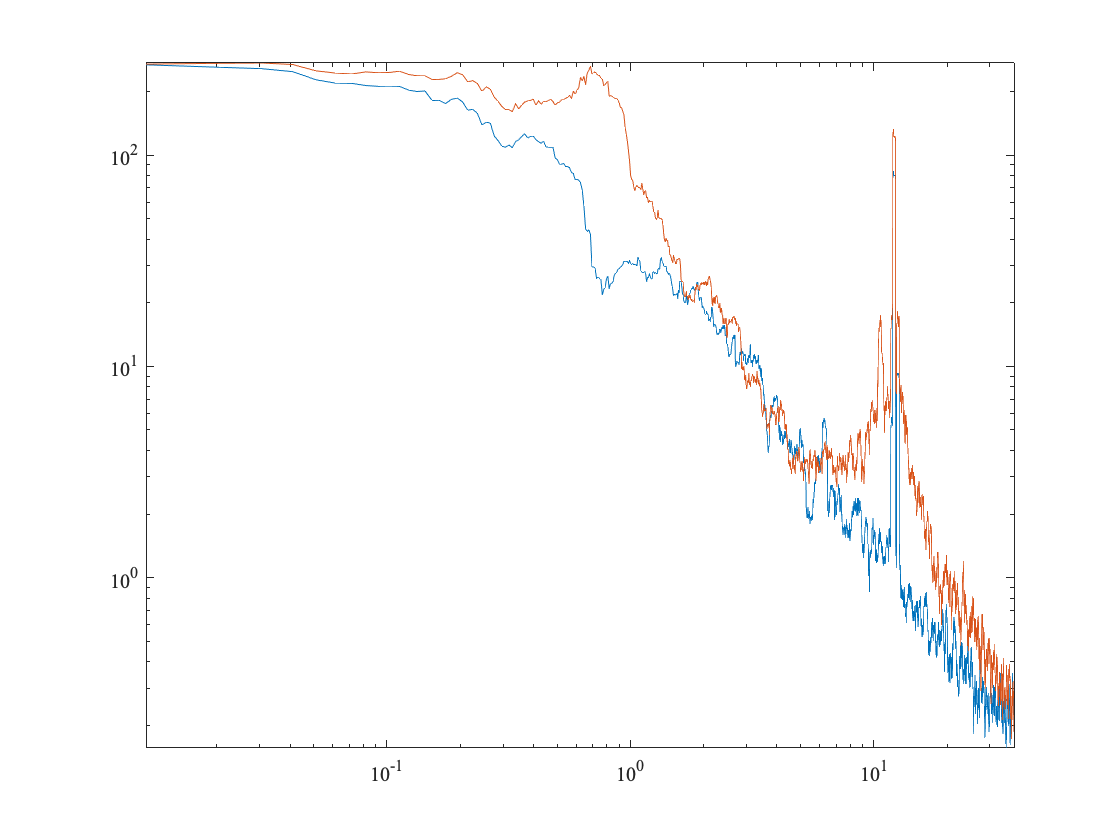First we will construct a narrowband filter to remove the intertial peak. Here we are using a new routine, wavetrans, which uses a special type of smoothing filter called a wavelet. Let's just run the code and then understand it afterward.
omega=2*pi*1200/length(cv);
cvo=frac(1,sqrt(2))*conj(wavetrans(conj(cv),{3,100,omega}));
cvr=cv-cvo;%Residual minus filtered version
[f,sppo,snno]=mspec(dt,cvo,psio);%Spectrum of filtered version
[f,sppr,snnr]=mspec(dt,cvr,psio);%Spectrum of residual
clf
%The next line returns the filter used by wavetrans
[psi,psif]=morsewave(length(cv),3,100,omega,'bandpass');
subplot(1,2,1),plot(f,[snn snnr])
axis(ax),xlog,ylog,xlim([max(f)/10 max(f)]),linestyle U T,flipx
hold on,plot(f,50*squared(psif(1:length(f))),'k') %get positive side only
subplot(1,2,2),plot(f,[spp sppr])
axis(ax),xlog,ylog,xlim([max(f)/10 max(f)]),linestyle U T
packfig(1,2,'columns') %pack the column subplots together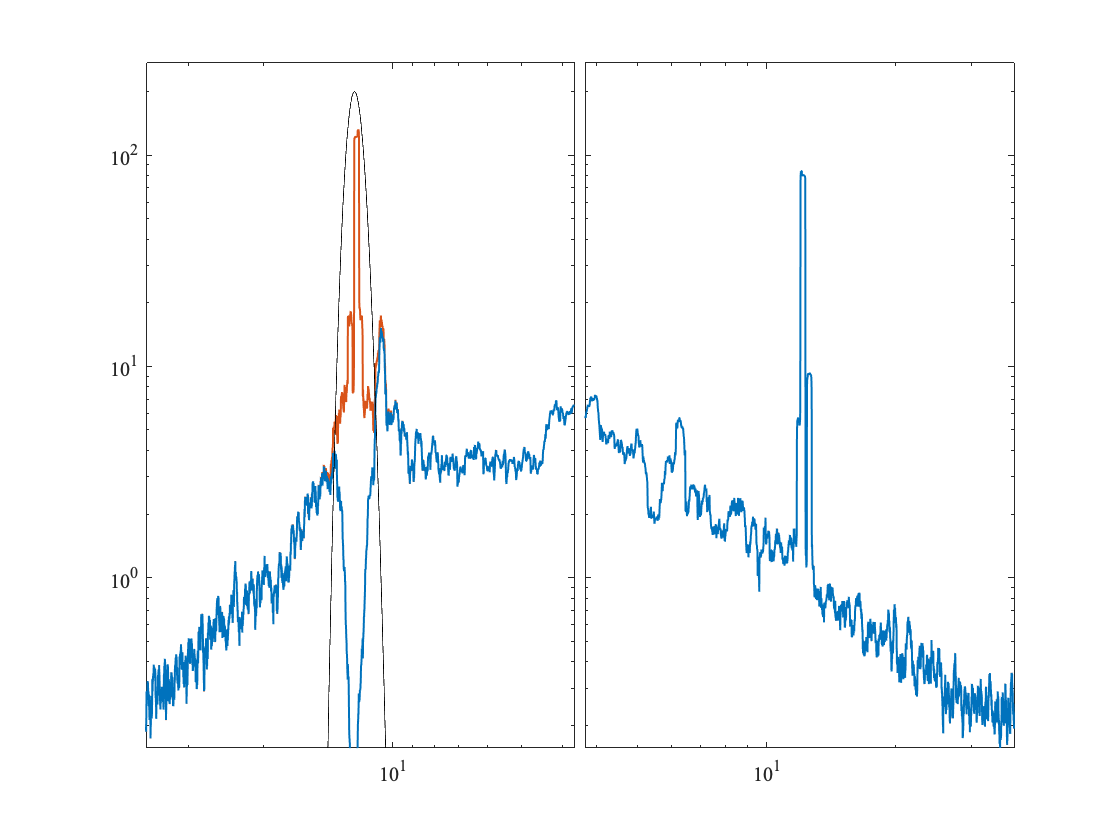As you can see, the negatively-rotating inertial peak has been removed, but the positive spectrum has not been touched at all. This means that the filtered version cvo has effectively isolated the inertial signal.
The wavelet transform essentially convolves a filter, the wavelet, with the original signal. This means we multiply the wavelet’s Fourier transform by the Fourier transform of the data. As the wavelet is a *narrowband* signal, this essentially shuts off all frequencies except those inside the band. Subtracting gives us the original signal with that band removed.
Wavetrans implements a bandpass filter with parameter specified by an argument in braces, which has the form
%{gamma,beta,omega}.
Here gamma is a parameter controlling the filter shape, beta is a parameter controlling the filter width in frequency, and omega is the filter center frequency in radians per sample interval. We will understand all of these more later.
Note, a very important feature of the wavelet filter is that it is one-sided. That is, if we tell it to implement a bandpass filter centred around frequency 10, we mean frequency +10 and not frequency -10. Only positively phasors will be affected and negatively rotating phasors.
To cause wavetrans to affect negative frequencies, as we wish to do here for the inertial filtering, we conjugate the input signal---which we saw will conjugate its Fourier transform but also flip positive and negative frequencies. Then to put the frequencies back, we also conjugate the output.
There is also a factor of 1/sqrt(2). This factor arises because there is a factor of sqrt(2) ambiguity in defining the amplitude of a real-valued versus a complex-valued sinusoid. Essentially we have defined a real-valued sinusoid to have amplitude one, with a consequence being that when we use wavetrans to form a bandpassed version of a complex valued signal, we need to divide by sqrt(2).
I chose the parameters as follows. Gamma is generally set to 3 for reasons we will see later. I found the frequency by noticing that the tidal peak was centered on about the 1200th Fourier frequency after calling plot(spp). The I picked beta by increasing it until it subjectively looked right.
Here is what the time series look like.
clf,
subplot(3,1,1),uvplot(yearfrac(num),cv),axis tight
subplot(3,1,2),uvplot(yearfrac(num),cvo),axis tight
hold on,plot(yearfrac(num),[abs(cvo) -abs(cvo)],'k','linewidth',2)
subplot(3,1,3),uvplot(yearfrac(num),cvr),axis tight
packfig(3,1,'rows')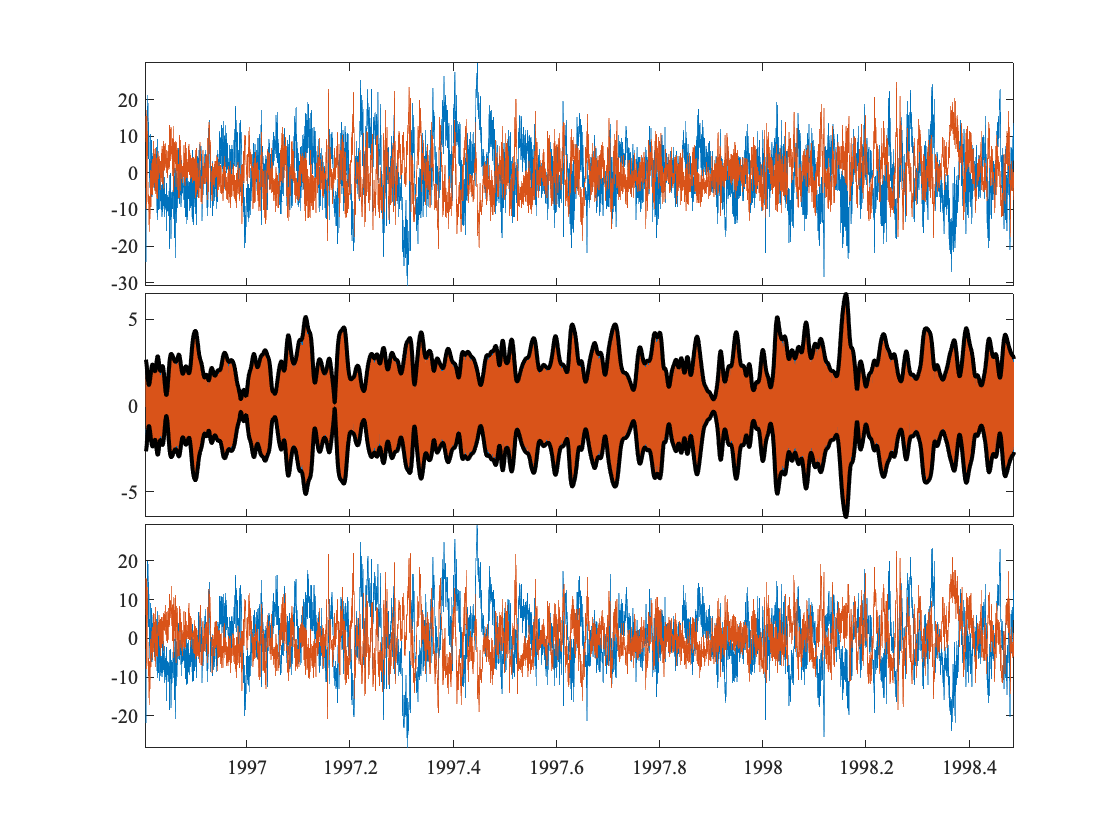Rather than just lowpassing, we have selectively removed only the inertial peak. A very useful facet of this analysis is that we now have the amplitude of our extracted signal, the inertial band in this case, as plotted with the heavy curve in the second panel. The blue curve is there but is not visible unless you zoom in.
We could also now proceed to look at the variance ellipses, statistics, etc, of the inertial band and residual separately. Combining simple statistics with bandpass filtering in this way can be very illuminating.
Next let's remove the tidal peak instead. This will be done by working with the positive and negative sides separately. However, for reference, code to do the same thing using the real and imaginary parts is also included, If one uses the real and imaginary parts of the data, there is no factor of sqrt(2). But since the wavelet is a one-sided bandpass, It will return something complex-valued if we give it a real-valued input. Consequently we need to take the real part of the both output variables.
cvo1=wavetrans(cv,{3,500,2*pi*1200/length(cv)});
cvo2=conj(wavetrans(conj(cv),{3,500,2*pi*1200/length(cv)}));
cvo=frac(1,sqrt(2))*(cvo1+cvo2);
%%This next bit is the same as the above
%[uplus,vplus]=wavetrans(real(cv),imag(cv),{3,500,2*pi*1200/length(cv)});
%cvo=real(uplus)+1i*real(vplus); %Note must take real part!
cvr=cv-cvo;
[f,sppo,snno]=mspec(dt,cvo,psio);
[f,sppr,snnr]=mspec(dt,cvr,psio);
%figure
subplot(1,2,1),plot(f,[snn snnr])
axis(ax),xlog,ylog,xlim([max(f)/10 max(f)]),linestyle U T,flipx
subplot(1,2,2),plot(f,[spp sppr])
axis(ax),xlog,ylog,xlim([max(f)/10 max(f)]),linestyle U T
packfig(1,2,'columns')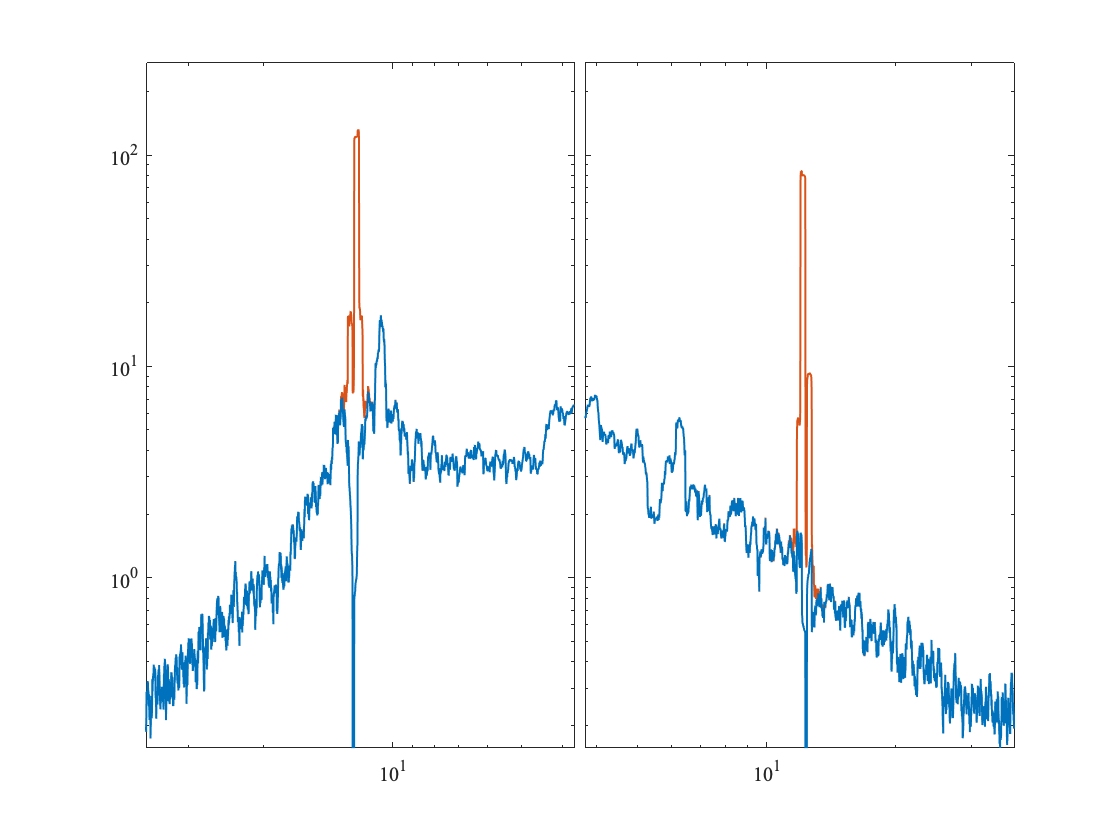We see that the tidal peak has indeed been removed from both sides.
Now that we have a general understanding of the wavelet transformer as implementing a one-sided bandpass, we will turn to the wavelet transform itself.

# Simple Wavelet Analysis

To begin with we will look at the downstream component of the m1244 mooring.
use m1244
dt=num(2)-num(1); %Sample rate in days
phi=angle(mean(cv(:,4))); %Find angle of downstream direction
u=real(rot(-phi)*cv(:,4)); %Just take the downstream component at first
gamma=3;beta=2; %Choose wavelet parameters
fs=morsespace(gamma,beta,length(u));%Specify wavelet frequency array
wu=wavetrans(u,{gamma,beta,fs}); %Take wavelet transform
clf,
h=wavespecplot(yearfrac(num),vfilt(u,24),dt./(fs/2/pi),wu);
axes(h(1)),axis tight
title('Wavelet Transform of Downstream Velocity')
axes(h(2)),ylabel('Period in Days')
hlines(1./(tidefreq('m2')*24/2/pi),'w--'),caxis([0 15])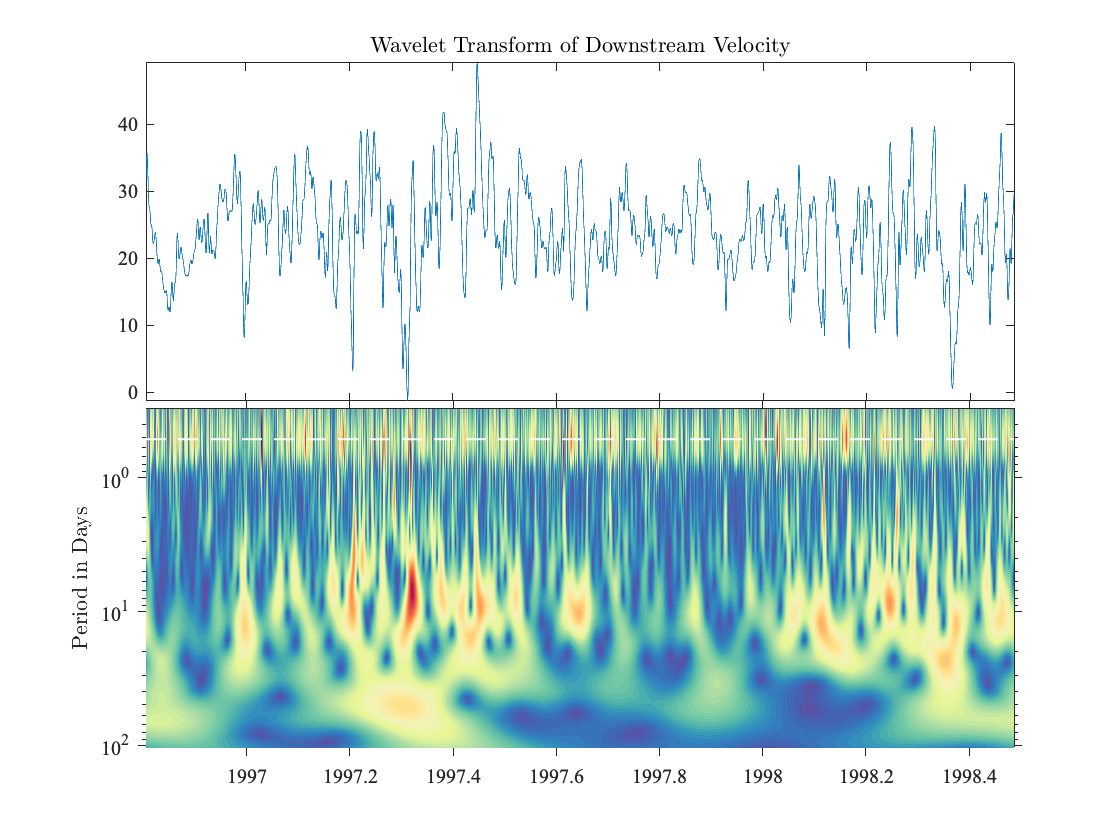This decomposes the variability as a function of both time and period (or equivalently, frequency). We will look more at how this works shortly. In the version of the wavelet transform used here, each horizontal band averages in time to an estimate of the Fourier spectrum at that frequency. In other words, we can think of the wavelet transform as in a sense unwrapping the spectrum across time.
Here, we see two major features. The first is an internal wave / tidal band, which is the stripe at the top of the figure. For reference this has been marked with the white dotted line, which shows the semidiurnal period of one half day, or a frequency of two cycles per day. The second major feature is a series of vertically elongated structures that have their maximum expression at around a period of 10 days. This is a very characteristic appearance of eddy-like variabillity. In general, wave-like variabilty tends to appear as a horizontal band, where time-localized features such as eddies tend to appear in the wavelet transform as vertically elongated features.
Thus, the main message here is that the variability seen in the spectrum arises from different structural causes---a wave-like aspect together with an eddy-like aspect. This is not apparent in the spectrum. While this is very useful in order to gain some impression of what the variability is like, in order to go further and say something quantitative about the variability takes a lot (by which I mean a huge amount) more work. Therefore most of the time we are satisfied with a qualitative or impressionistic interpretation of the wavelet transform. For certain problems, we can create (or apply) very powerful quantitative methods based on the wavelet transform, but we have to proceed carefully because they take a long time to learn.
Before continuing to understand the interpretation of the wavelet transform in more detail, let's first look at the steps involved in computing it.

# Computing the Wavelet Transform

What is a wavelet? A wavelet is simply an oscillatory, time-localized function. A good example is a complex exponential multiplied by a Gaussian; this is an archetypal wavelet called the Morlet wavelet. The complex exponential generates the oscillation, while the Gaussian controls the time localization. At every time, we will compare the wavelet with the time series in order to meaure how much local oscillatory variabilty there is at that time and at a particular frequency. Then we stretch out the wavelet, thus lowering its frequency (by the scaling theorem), and we again compare the wavelet with the time series at each moment. Thus, the wavelet is used as a sort of measuring stick for quantifying oscillatory variability.
The first question to ask is which wavelet to use. This use to be a complicated question, as there were many different types of wavelets that had been proposed, and it was not clear which were superior for which purposes. This made it difficult to even know where to start.
Myself and colleague, Sofia Olhede, looked into this problem in this short paper. We found that all the many types of wavelets in the literature were essentially special cases of a single, simple, and very broad family. The name of this family of wavelets is the Morse wavelets. Within that family, we were able to answer the question of what wavelets are ‘best’ in a certain sense. The Morse wavelets are controlled by two parameters, known as β (beta) and γ (gamma). So determining which wavelets are ‘best’ is about determining the optimal settting of these two parameters.
We showed that choosing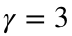is desirable because it gives wavelets having a high degree of symmetry in the frequency domain, a property that tends to lead to good performance. Varying β with γ held fixed then essential varies the degree of ‘wiggliness’, or more formally the bandwidth, of the wavelet. Increasing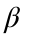leads to wavelets which are more oscillatory and therefore have a smaller, or tighter, bandwidth in the frequency domain. Generally in wavelet analysis, we are interested in choosing β to be small, as we are interested in wavelets that are highly time-localized as opposed to frequency-localized. With, β should be no smaller than one; otherwise the wavelets are too time-localized and no longer behave like oscillations.
The question of which wavelet to use is therefore generally answered as follows. Using the Morse wavelets with, and varyuntil you find a value that seems to lead to the best visiual representation of the variability in your time series. That's it. In other words, β is a choice that you can make depending upon what you want to look at in the time series. Essentially, β is controlling the trade-off of time resolution against frequency resolution.
Knowing how to choose a wavelet makes your life a lot simpler. Matlab developers agreed. They read our paper, then threw out all their old wavelet code, and rewrote it code according to our recommendations. Look at the references at the bottom of this Matlab help page. So the wavelets that we are using here are also now the default wavelets in Matlab. Unlike Matlab's version, however, you don't have to pay \$1000 to use my code. It's free.
I should mention that here we are talking about continuous wavelets, meaning wavelets defined on continuous time. Continuous wavelets are what is used in analyzing signals. There are also discrete wavelets, which are totally different. These are commonly used in, for example, compressing a signal into fewer bits of information, as one compresses an image. I never use discrete wavelets, so whenever I say ‘wavelet’ I always mean ‘continuous wavelet’.
Let's continue examining the code above.
gamma=3;beta=2; %Choose wavelet parameters
fs=morsespace(gamma,beta,length(u));%Specify wavelet frequency array
wu=wavetrans(u,{gamma,beta,fs}); %Take wavelet transform
clf,